This article has been archived and is no longer being updated. It may not work with the most recent OS versions.

# Procedural Level Generation in Games Tutorial: Part 1

A tutorial on procedural level generation using the Drunkard Walk algorithm.

#### Version

• Other, Other, Other

Note from Ray: This is a brand new Sprite Kit tutorial released as part of the iOS 7 Feast. Enjoy!

Most games you play have carefully designed levels that always remain the same. Experienced players know exactly what happens at any given time, when to jump and what button to press. While this is not necessarily a bad thing, it does to some extent reduce the game’s lifespan with a given player. Why play the same level over and over again?

One way to increase your game’s replay value is to allow the game to generate its content programmatically – also known as adding procedurally generated content.

In this tutorial, you will learn to create tiled dungeon-like levels using an algorithm called the Drunkard Walk. You will also create a reusable `Map` class with several properties for controlling a level’s generation.

This tutorial uses Sprite Kit, a framework introduced with iOS 7. You will also need Xcode 5. If you are not already familiar with Sprite Kit, I recommend you read the Sprite Kit Tutorial for Beginners on this site. For readers who are not yet ready to switch to Sprite Kit, fear not. You can easily rewrite the code in this tutorial to use Cocos2d.

## Getting Started

Before getting started, let’s clear up one possible misconception: procedural should not be confused with random. Random means that you have little control over what happens, which should not be the case in game development.

Even in procedurally generated levels, your player should be able to reach the exit. What would be the fun of playing an endless runner like Canabalt if you got to a gap between buildings that would be impossible to jump? Or playing a platformer where the exit is in a place you cannot reach? In this sense, it might be even harder to design a procedurally generated level than to carefully craft your level in Tiled.

I assume, being the bad-ass coder that you are, that you scoff at such cautionary statements. To get started, download the starter project for this tutorial. Once downloaded, unzip the file and open the project in Xcode, and build and run. You should now see a screen similar to this:

The starter project contains the basic building blocks of the game, including all necessary artwork, sound effects and music. Take note of the following important classes:

• `Map`: Creates a basic 10×10 square that functions as the level for the game.
• `MapTiles`: A helper class that manages a 2D grid of tiles. I will explain this class later in the tutorial.
• `DPad`: Provides a basic implementation of a joystick to control the player’s character, a cat.
• `MyScene`: Sets up the Sprite Kit scene and processes game logic.

Spend a few moments getting familiar with the code in the starter project before moving on. There are comments to help you understand how the code works. Also, try playing the game by using the DPad at the bottom-left corner to move the cat to the exit. Notice how the start and exit points change every time the level begins.

## The Beginnings of a New Map

If you played the starter game more than once, you probably discovered that the game isn’t very fun. As Jordan Fisher writes in GamaSutra, game levels, especially procedurally generated ones, need to nail these three criteria to be successful:

• Feasibility: Can you beat the level?
• Interesting design: Do you want to beat it?
• Skill level: Is it a good challenge?

Your current level fails two of these three criteria: The design is not very interesting, as the outer perimeter never changes, and it is too easy to win, as you can always see where the exit is when the level starts. Hence, to make the level more fun, you need to generate a better dungeon and make the exit harder to find.

The first step is to change the way you generate the map. To do so, you’ll delete the `Map` class and replace it with a new implementation.

Select Map.h and Map.m in the Project Navigator, press Delete and then select Move to Trash.

Next go to File\New\New File…, choose the iOS\Cocoa Touch\Objective-C class and click Next. Name the class Map, make it a Subclass of SKNode and click Next. Make sure the ProceduralLevelGeneration target is selected and click Create.

Open Map.h and add the following code to the `@interface` section:

```@property (nonatomic) CGSize gridSize;
@property (nonatomic, readonly) CGPoint spawnPoint;
@property (nonatomic, readonly) CGPoint exitPoint;

+ (instancetype) mapWithGridSize:(CGSize)gridSize;
- (instancetype) initWithGridSize:(CGSize)gridSize;
```

This is the interface that `MyScene` expects for the `Map` class. You specify here where to spawn the player and exit, and create some initializers to construct the class given a certain size.

Implement these in Map.m by adding this code to the `@implementation` section:

```+ (instancetype) mapWithGridSize:(CGSize)gridSize
{
return [[self alloc] initWithGridSize:gridSize];
}

- (instancetype) initWithGridSize:(CGSize)gridSize
{
if (( self = [super init] ))
{
self.gridSize = gridSize;
_spawnPoint = CGPointZero;
_exitPoint = CGPointZero;
}
return self;
}
```

Here you add a stub implementation that simply sets the player spawn and exit points to `CGPointZero`. This will allow you to have a simple starting point – you’ll fill these out to be more interesting later.

Build and run, and you should see the following: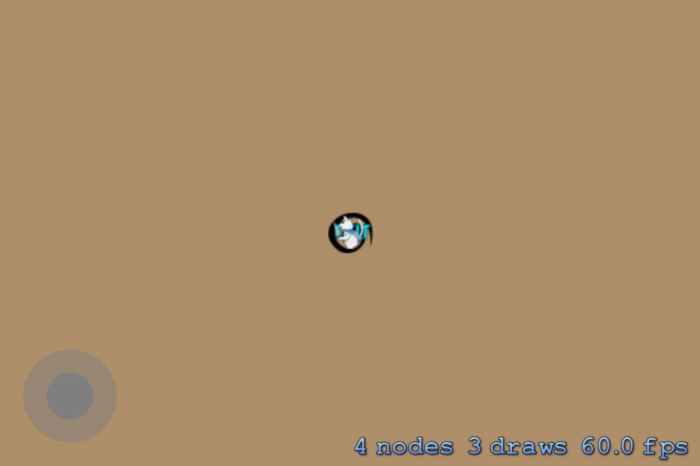Gone are the borders of the map and the feline hero gets sucked right into the exit, making the game unplayable – or really, really easy if you are a glass-half-full kind of person. Not really the a-maze-ing (pun intended) game you were hoping for, right? Well, time to put down some floors. Enter the Drunkard Walk algorithm.

## The Drunkard Walk Algorithm

The Drunkard Walk algorithm is a kind of random walk and one of the simplest dungeon generation algorithms around. In its simplest implementation, the Drunkard Walk algorithm works as follows:

1. Choose a random start position in a grid and mark it as a floor.
2. Pick a random direction to move (Up, Down, Left or Right).
3. Move in that direction and mark the position as a floor, unless it already is a floor.
4. Repeat steps 2 and 3 until a desired number of floors have been placed in the grid.

Nice and simple, eh? Basically, it is a loop that runs until a desired number of floors have been placed in the map. To allow the map generation to be as flexible as possible, you will start implementing the algorithm by adding a new property to hold the number of tiles to generate.

Open Map.h and add the following property:

`@property (nonatomic) NSUInteger maxFloorCount;`

Next, open Map.m and add the following method:

```- (void) generateTileGrid
{
CGPoint startPoint = CGPointMake(self.gridSize.width / 2, self.gridSize.height / 2);

NSUInteger currentFloorCount = 0;

while ( currentFloorCount < self.maxFloorCount )
{
currentFloorCount++;
}
}
```

The above code begins to implement step 1 in the basic Drunkard Walk algorithm loop, but there is one significant difference. Can you spot it?

[spoiler title="Solution"]`startPoint` is defaulted to the center of the grid instead of a random position. You do this to prevent the algorithm from butting up against the edges and getting stuck. More about that in the second part of the tutorial.[/spoiler]

`generateTileGrid` begins by setting a start position and then enters a loop that runs until the `currentFloorCount` is equal to the desired number of floors defined by the `maxFloorCount` property.

When you initialize a `Map` object, you should invoke `generateTileGrid` to ensure that you create the grid. So, add the following code to `initWithGridSize:` in Map.m, after the `_exitPoint = CGPointZero` line:

`[self generateTileGrid];`

Build and run to make sure the game compiles as expected. Nothing has changed since the last run. The cat is still sucked into the exit and there are still no walls. You still need to write the code to generate the floor, but before you do that, you need to understand the `MapTiles` helper class.

## Managing the Tile Grid

The `MapTiles` class is essentially a wrapper for a dynamic C array that will manage a 2D grid for the `Map` class.

Note: If you're wondering why I choose to use a C array instead of an `NSMutableArray`, it comes down to personal preference. I generally do not like boxing primitive data types like integers into objects and then unboxing them again to use them, and since the `MapTiles` grid is just an array of integers, I prefer a C array.

The `MapTiles` class is already in your project. If you've taken a glance through and feel you understand how it works well, feel free to skip ahead to the next section, Generating the Floor.

But if you're unsure about how it works, keep reading to learn how to recreate it step-by-step, and I'll explain how it works along the way.

To start, select MapTiles.h and MapTiles.m in the Project Navigator, press Delete and then select Move to Trash.

Go to File\New\File..., choose the iOS\Cocoa Touch\Objective-C class and click Next. Name the class MapTiles, make it a subclass of NSObject and click Next. Be sure the ProceduralLevelGeneration target is selected and click Create.

In order to make it easy to identify the type of tile, add this enum below the `#import` statement in MapTiles.h:

```typedef NS_ENUM(NSInteger, MapTileType)
{
MapTileTypeInvalid = -1,
MapTileTypeNone = 0,
MapTileTypeFloor = 1,
MapTileTypeWall = 2,
};
```

If later on you want to extend the `MapTiles` class with further tile types, you should put those in this `MapTileType` enum.

Note: Notice the integer values you assign to each of the enums. They weren't picked at random. Look in the tiles.atlas texture atlas and click the 1.png file, and you will see that it is the texture for the floor just as `MapTileTypeFloor` has a value of 1. This makes it easy to convert the 2D grid array into tiles later on.

Open MapTiles.h and add the following properties and method prototypes between `@interface` and `@end`:

```@property (nonatomic, readonly) NSUInteger count;
@property (nonatomic, readonly) CGSize gridSize;

- (instancetype) initWithGridSize:(CGSize)size;
- (MapTileType) tileTypeAt:(CGPoint)tileCoordinate;
- (void) setTileType:(MapTileType)type at:(CGPoint)tileCoordinate;
- (BOOL) isEdgeTileAt:(CGPoint)tileCoordinate;
- (BOOL) isValidTileCoordinateAt:(CGPoint)tileCoordinate;
```

You've added two read-only properties: `count` provides the total number of tiles in the grid and `gridSize` holds the width and height of the grid in tiles. You'll find these properties handy later on. I'll explain the five methods as you implement the code.

Next, open MapTiles.m and add the following class extension right above the `@implementation` line:

```@interface MapTiles ()
@property (nonatomic) NSInteger *tiles;
@end
```

This code adds a private property `tiles` to the class. This is a pointer to the array that holds information about the tile grid.

Now implement initWithGridSize: in MapTiles.m after the `@implementation` line:

```- (instancetype) initWithGridSize:(CGSize)size
{
if (( self = [super init] ))
{
_gridSize = size;
_count = (NSUInteger) size.width * size.height;
self.tiles = calloc(self.count, sizeof(NSInteger));
NSAssert(self.tiles, @"Could not allocate memory for tiles");
}
return self;
}
```

You initialize the two properties in `initWithGridSize:`. Since the total number of tiles in the grid is equal to the width of the grid multiplied by the grid height, you assign this value to the `count` property. Using this count, you allocate the memory for the tiles array with `calloc`, which ensures all variables in the array are initialized to 0, equivalent to the enumerated variable `MapTileTypeNone`.

As ARC will not manage memory allocated using `calloc` or `malloc`, you should release the memory whenever you deallocate the `MapTiles` object. Before `initWithGridSize:` but after `@implementation`, add the `dealloc` method:

```- (void) dealloc
{
if ( self.tiles )
{
free(self.tiles);
self.tiles = nil;
}
}
```

`dealloc` frees the memory when you deallocate an object and resets the `tiles` property pointer to avoid it pointing to an array that no longer exists in memory.

Apart from the construction and deconstruction, the `MapTiles` class also has a few helper methods for managing tiles. But before you start implementing these methods, you need to understand how the tiles array exists in memory versus how it is organized in a grid.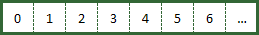Figure 1: How `calloc` organizes the variables in memory. Each number is the index of the variable in memory.

When you allocate memory for the tiles using `calloc`, it reserves n bytes for each array item, depending on the data type, and puts them end-to-end in a flat structure in memory (see figure 1).

This organization of tiles is hard to work with in practice. It is much easier to find a tile by using an (x,y) pair of coordinates, as illustrated in Figure 2, so that is how the `MapTiles` class should organize the tile grid.

Thankfully, it is very easy to calculate the index of a tile in memory from an (x,y) pair of coordinates since you know the size of the grid from the `gridSize` property. The numbers outside the square in Figure 2 illustrate the x- and y-coordinates, respectively. For example, the (x,y) coordinates (1,2) in the grid will be index 9 of the array. You calculate this using the formula:

index in memory = y * gridSize.width + x

With this knowledge, you can start implementing a method that will calculate an index from a pair of grid coordinates. For convenience, you will also create a method to ensure the grid coordinates are valid.

In MapTiles.m, add the following new methods:

```- (BOOL) isValidTileCoordinateAt:(CGPoint)tileCoordinate
{
return !( tileCoordinate.x < 0 ||
tileCoordinate.x >= self.gridSize.width ||
tileCoordinate.y < 0 ||
tileCoordinate.y >= self.gridSize.height );
}

- (NSInteger) tileIndexAt:(CGPoint)tileCoordinate
{
if ( ![self isValidTileCoordinateAt:tileCoordinate] )
{
NSLog(@"Not a valid tile coordinate at %@", NSStringFromCGPoint(tileCoordinate));
return MapTileTypeInvalid;
}
return ((NSInteger)tileCoordinate.y * (NSInteger)self.gridSize.width + (NSInteger)tileCoordinate.x);
}
```

`isValidTileCoordinateAt:` tests if a given pair of coordinates is within the bounds of the grid. Notice how the method checks to see if it is outside of the bounds and then returns the opposite result, so if the coordinates are outside the bounds, it returns `NO`, and if they are not outside of the bounds, it returns `YES`. This is faster than checking if the coordinates are within the bounds, which would require the conditions to be AND-ed together instead of OR-ed.

`tileIndexAt:` uses the equation discussed above to calculate an index from a pair of coordinates, but before doing this, it tests if the coordinates are valid. If not, it returns `MapTileTypeInvalid`, which has a value of -1.

With the math in place, it is now possible to easily create the methods to return or set the tile type. So, add the following two methods after `initWithGridSize:` in MapTiles.m:

```- (MapTileType) tileTypeAt:(CGPoint)tileCoordinate
{
NSInteger tileArrayIndex = [self tileIndexAt:tileCoordinate];
if ( tileArrayIndex == -1 )
{
return MapTileTypeInvalid;
}
return self.tiles[tileArrayIndex];
}

- (void) setTileType:(MapTileType)type at:(CGPoint)tileCoordinate
{
NSInteger tileArrayIndex = [self tileIndexAt:tileCoordinate];
if ( tileArrayIndex == -1 )
{
return;
}
self.tiles[tileArrayIndex] = type;
}
```

The two methods calculate the index from the pair of coordinates passed using the `tileIndexAt:` method you just added and then either set or return the `MapTileType` from the `tiles` array.

Last but not least, add a method to determine if a given pair of tile coordinates is at the edge of the map. You'll later use this method to ensure you do not place any floors at the edge of the grid, thereby making it impossible to encapsulate all floors behind walls.

```- (BOOL) isEdgeTileAt:(CGPoint)tileCoordinate
{
return ((NSInteger)tileCoordinate.x == 0 ||
(NSInteger)tileCoordinate.x == (NSInteger)self.gridSize.width - 1 ||
(NSInteger)tileCoordinate.y == 0 ||
(NSInteger)tileCoordinate.y == (NSInteger)self.gridSize.height - 1);
}
```

Referring to Figure 2 above, notice that border tiles would be any tile with an x-coordinate of 0 or `gridSize.width – 1`, since the grid indices are zero-based. Equally, an y-coordinate of 0 or `gridSize.height – 1` would be a border tile.

Finally, when testing it's nice to be able to see what your procedural generation is actually generating. Add the following implementation of `description`, which will output the grid to the console for easy debugging:

```- (NSString *) description
{
NSMutableString *tileMapDescription = [NSMutableString stringWithFormat:@"<%@ = %p | \n",
[self class], self];

for ( NSInteger y = ((NSInteger)self.gridSize.height - 1); y >= 0; y-- )
{
[tileMapDescription appendString:[NSString stringWithFormat:@"[%i]", y]];

for ( NSInteger x = 0; x < (NSInteger)self.gridSize.width; x++ )
{
[tileMapDescription appendString:[NSString stringWithFormat:@"%i",
[self tileTypeAt:CGPointMake(x, y)]]];
}
[tileMapDescription appendString:@"\n"];
}
return [tileMapDescription stringByAppendingString:@">"];
}
```

This method simply loops through the grid to create a string representation of the tiles.

That was a lot of text and code to take in, but what you've built will make the procedural level generation much easier, since you can now abstract the grid handling from the level generation. Now it's time to lay down some ground.

## Generating the Floor

You're going to place ground or floor tiles procedurally in the map using the Drunkard Walk algorithm discussed above. In Map.m, you already implemented part of the algorithm so that it finds a random start position (step 1) and loops a desired number of times (step 4). Now you need to implement steps 2 and 3 to generate the actual floor tiles within the loop you created.

To make the `Map` class a bit more flexible, you'll start by adding a dedicated method to generate a procedural map. This will also be handy if you later need to regenerate the map.

Open Map.h and add the following method declaration to the interface:

```- (void) generate;
```

In Map.m, add the following import to the top of the file:

```#import "MapTiles.h"
```

Add the following code right above the `@implementation` line:

```@interface Map ()
@property (nonatomic) MapTiles *tiles;
@end
```

The class extension holds one private property, which is a pointer to a `MapTiles` object. You'll use this object for easy grid handling in the map generation. You're keeping it private since you don't want to change the `MapTiles` object from outside the `Map` class.

Next, implement the `generate` method in Map.m:

```- (void) generate
{
self.tiles = [[MapTiles alloc] initWithGridSize:self.gridSize];
[self generateTileGrid];
}
```

First the method allocates and initializes a `MapTiles` object, then it generates a new tile grid by calling `generateTileGrid`.

In Map.m, go to `initWithGridSize:` and delete this line:

```[self generateTileGrid];
```

You deleted that line because map generation should no longer occur immediately when you create a `Map` object.

It's time to add the code to generate the floor of the dungeon. Do you remember the remaining steps of the Drunkard Walk algorithm? You choose a random direction and then place a floor at the new coordinates.

The first step is to add a convenience method to provide a random number between two values. Add the following method in Map.m:

```- (NSInteger) randomNumberBetweenMin:(NSInteger)min andMax:(NSInteger)max
{
return min + arc4random() % (max - min);
}
```

You'll use this method to return a random number between min and max, both inclusive.

Return to `generateTileGrid` and replace its contents with the following:

```CGPoint startPoint = CGPointMake(self.tiles.gridSize.width / 2, self.tiles.gridSize.height / 2);
// 1
[self.tiles setTileType:MapTileTypeFloor at:startPoint];
NSUInteger currentFloorCount = 1;
// 2
CGPoint currentPosition = startPoint;
while ( currentFloorCount < self.maxFloorCount )
{
// 3
NSInteger direction = [self randomNumberBetweenMin:1 andMax:4];
CGPoint newPosition;
// 4
switch ( direction )
{
case 1: // Up
newPosition = CGPointMake(currentPosition.x, currentPosition.y - 1);
break;
case 2: // Down
newPosition = CGPointMake(currentPosition.x, currentPosition.y + 1);
break;
case 3: // Left
newPosition = CGPointMake(currentPosition.x - 1, currentPosition.y);
break;
case 4: // Right
newPosition = CGPointMake(currentPosition.x + 1, currentPosition.y);
break;
}
//5
if([self.tiles isValidTileCoordinateAt:newPosition] &&
![self.tiles isEdgeTileAt:newPosition] &&
[self.tiles tileTypeAt:newPosition] == MapTileTypeNone)
{
currentPosition = newPosition;
[self.tiles setTileType:MapTileTypeFloor at:currentPosition];
currentFloorCount++;
}
}
// 6
_exitPoint = currentPosition;
// 7
NSLog(@"%@", [self.tiles description]);
```

This is what the code is doing:

1. It marks the tile at coordinates `startPoint` in the grid as a floor tile and therefore initializes `currentFloorCount` with a count of 1.
2. `currentPosition` is the current position in the grid. The code initializes it to the `startPoint` coordinates where the Drunkard Walk algorithm will start.
3. Here the code chooses a random number between 1 and 4, providing a direction to move (1 = UP, 2 = DOWN, 3 = LEFT, 4 = RIGHT).
4. Based on the random number chosen in the above step, the code calculates a new position in the grid.
5. If the newly calculated position is valid and not an edge, and does not already contain a tile, this part adds a floor tile at that position and increments `currentFloorCount` by 1.
6. Here the code sets the last tile placed to the exit point. This is the goal of the map.
7. Lastly, the code prints the generated tile grid to the console.

Build and run. The game runs with no visible changes, but it fails to write the tile grid to the console. Why is that?

[spoiler title="Solution"]You never call `generate` on the `Map` class during `MyScene` initialization. Therefore, you created the map object but don't actually generate the tiles.[/spoiler]

To fix this, go to MyScene.m and in `initWithSize:`, replace the line `self.map = [[Map alloc] init]` with the following:

```self.map = [[Map alloc] initWithGridSize:CGSizeMake(48, 48)];
self.map.maxFloorCount = 64;
[self.map generate];
```

This generates a new map with a grid size of 48 by 48 tiles and a desired maximum floor count of 64. Once you set the `maxFloorCount` property, you generate the map.

Build and run again, and you should see an output that resembles something similar to, but probably not exactly like (remember, it's random), the following:HOORAY!! You have generated a procedural level. Pat yourself on the back and get ready to show your masterpiece on the big – or small – screen.

## Converting a Tile Grid into Tiles

Plotting your level in the console is a good way to debug your code but a poor way to impress your player. The next step is to convert the grid into actual tiles.

The starter project already includes a texture atlas containing the tiles. To load the atlas into memory, add a private property to the class extension of Map.m, as well as a property to hold the size of a tile:

```@property (nonatomic) SKTextureAtlas *tileAtlas;
@property (nonatomic) CGFloat tileSize;
```

Initialize these two properties in `initWithGridSize:`, just after setting the value of `_exitPoint`:

```self.tileAtlas = [SKTextureAtlas atlasNamed:@"tiles"];

NSArray *textureNames = [self.tileAtlas textureNames];
SKTexture *tileTexture = [self.tileAtlas textureNamed:(NSString *)[textureNames firstObject]];
self.tileSize = tileTexture.size.width;
```

After loading the texture atlas, the above code reads the texture names from the atlas. It uses the first name in the array to load a texture and stores that texture's width as `tileSize`. This code assumes textures in the atlas are squares (same width and height) and are all of the same size.

Note: Using a texture atlas reduces the number of draw calls necessary to render the map. Every draw call adds overhead to the system because Sprite Kit has to perform extra processing to set up the GPU for each one. By using a single texture atlas, the entire map may be drawn in as few as a single draw call. The exact number will depend on several things, but in this app, those won't come into play. To learn more, check out Chapter 25 in iOS Games by Tutorials, Performance: Texture Atlases.

Still inside Map.m, add the following method:

```- (void) generateTiles
{
// 1
for ( NSInteger y = 0; y < self.tiles.gridSize.height; y++ )
{
for ( NSInteger x = 0; x < self.tiles.gridSize.width; x++ )
{
// 2
CGPoint tileCoordinate = CGPointMake(x, y);
// 3
MapTileType tileType = [self.tiles tileTypeAt:tileCoordinate];
// 4
if ( tileType != MapTileTypeNone )
{
// 5
SKTexture *tileTexture = [self.tileAtlas textureNamed:[NSString stringWithFormat:@"%i", tileType]];
SKSpriteNode *tile = [SKSpriteNode spriteNodeWithTexture:tileTexture];
// 6
tile.position = tileCoordinate;
// 7
}
}
}
}
```

`generateTiles` converts the internal tile grid into actual tiles by:

1. Two `for` loops, one for x and one for y, iterate through each tile in the grid.
2. This converts the current x- and y-values into a `CGPoint` structure for the position of the tile within the grid.
3. Here the code determines the type of tile at this position within the grid.
4. If the tile type is not an empty tile, then the code proceeds with creating the tile.
5. Based on the tile type, the code loads the respective tile texture from the texture atlas and assigns it to a `SKSpriteNode` object. Remember that the tile type (integer) is the same as the file name of the texture, as explained earlier.
6. The code sets the position of the tile to the tile coordinate.
7. Then it adds the created tile node as a child of the map object. This is done to ensure proper scrolling by grouping the tiles to the map where they belong.

Finally, make sure the grid is actually turned into tiles by inserting the following line into the `generate` method in Map.m, after `[self generateTileGrid]`:

```[self generateTiles];
```

Build and run — but the result is not as expected. The game incorrectly places the tiles in a big pile, as illustrated here: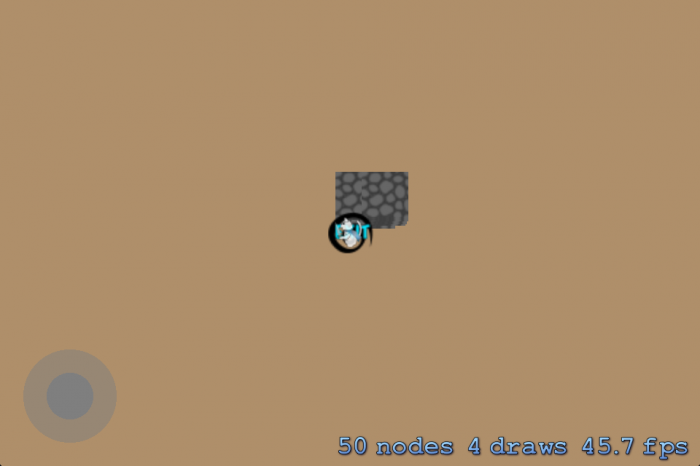The reason is straightforward: When positioning the tile, the current code sets the tile's position to the position within the internal grid and not relative to screen coordinates.

You need a new method to convert grid coordinates into screen coordinates, so add the following to Map.m:

```- (CGPoint) convertMapCoordinateToWorldCoordinate:(CGPoint)mapCoordinate
{
return CGPointMake(mapCoordinate.x * self.tileSize,  (self.tiles.gridSize.height - mapCoordinate.y) * self.tileSize);
}
```

By multiplying the grid (map) coordinate by the tile size, you calculate the horizontal position. The vertical position is slightly more complicated. Remember that the coordinates (0,0) in Sprite Kit represent the bottom-left corner. In the tile grid, the position of (0,0) is the top-left corner (see Figure 2 above). Hence, in order to correctly position the tile, you need to invert its vertical placement. You do this by subtracting the y-position of the tile in the grid by the total height of the grid and multiplying it by the tile size.

Revisit `generateTiles` and change the line that sets `tile.position` to the following:

```tile.position = [self convertMapCoordinateToWorldCoordinate:CGPointMake(tileCoordinate.x, tileCoordinate.y)];
```

Also, change the line that sets `_exitPoint` in `generateTileGrid` to the following:

```_exitPoint = [self convertMapCoordinateToWorldCoordinate:currentPosition];
```

Build and run – oh no, where did the tiles go?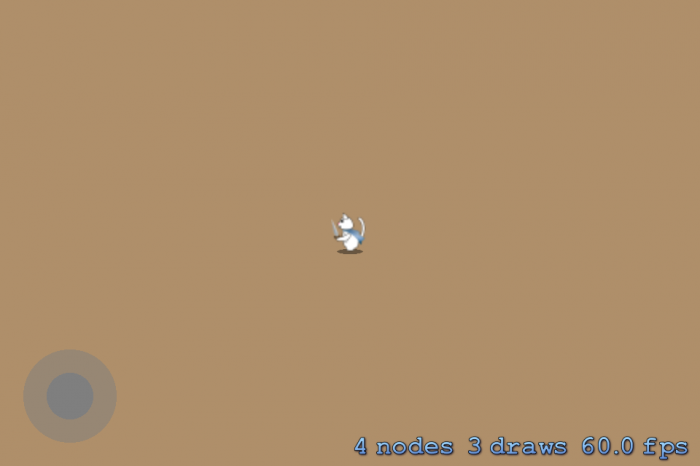Well, they are still there – they're just outside the visible area. You can easily fix this by changing the player's spawn position. You will apply a simple yet effective strategy where you set the spawn point to the position of the `startPoint` in `generateTileGrid`.

Go to `generateTileGrid` and add the following line at the very bottom of the method:

```_spawnPoint = [self convertMapCoordinateToWorldCoordinate:startPoint];
```

The spawn point is the pair of screen coordinates where the game should place the player at the beginning of the level. Hence, you calculate the world coordinates from the grid coordinates.

Build and run, and take the cat for a walk around the procedural world. Maybe you will even find the exit?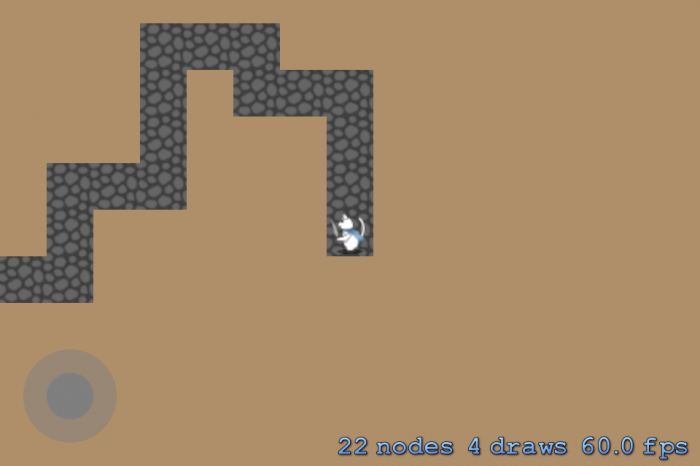Try playing around with different grid sizes and max number of floor tiles to see how it affects the map generation.

One obvious issue now is that the cat can stray from the path. And we all know what happens when cats stray, right? All the songbirds of the world shiver. So, time to put up some walls.

Open Map.m and add the following method:

```- (void) generateWalls
{
// 1
for ( NSInteger y = 0; y < self.tiles.gridSize.height; y++ )
{
for ( NSInteger x = 0; x < self.tiles.gridSize.width; x++ )
{
CGPoint tileCoordinate = CGPointMake(x, y);

// 2
if ( [self.tiles tileTypeAt:tileCoordinate] == MapTileTypeFloor )
{
for ( NSInteger neighbourY = -1; neighbourY < 2; neighbourY++ )
{
for ( NSInteger neighbourX = -1; neighbourX < 2; neighbourX++ )
{
if ( !(neighbourX == 0 && neighbourY == 0) )
{
CGPoint coordinate = CGPointMake(x + neighbourX, y + neighbourY);

// 3
if ( [self.tiles tileTypeAt:coordinate] == MapTileTypeNone )
{
[self.tiles setTileType:MapTileTypeWall at:coordinate];
}
}
}
}
}
}
}
}
```
1. The strategy applied by `generateWalls` is to first loop through each tile of the grid.
2. It does this until it identifies a floor tile (`MapTileTypeFloor`).
3. It then checks the surrounding tiles and marks these as walls (`MapTileTypeWall`) if no tile is placed there already (`MapTileTypeNone`).

The inner `for` loops (after `//2`) might seem a bit strange at first. It looks at each tile that surrounds the tile at coordinate (x,y). Take a peek at Figure 3 and see how the tiles you want are one less, equal to, and one more than the original index. The two `for` loop gives just that, starting at -1 and looping through to +1. Adding one of these integers to the original index inside the `for` loop, you find each neighbor.

What if the tile you're checking is at the border of the grid? In that case, this check would fail, as the index would be invalid, correct?

Yes, but luckily this situation is mitigated by the `tileTypeAt:` method on the `MapTiles` class. If an invalid coordinate is sent to `tileTypeAt:`, the method will return a `MapTileTypeInvalid` value. Consider the line after `//3` in `generateWalls` and notice it only changes the tile to a wall tile if the returned tile type is `MapTileTypeNone`.

To generate the wall tiles, go back to `generate` in Map.m and add the following line of code after `[self generateTileGrid]` and before `[self generateTiles]`:

```[self generateWalls];
```

Build and run. You should now see wall tiles surrounding the floor tiles. Try moving the cat around – notice anything strange?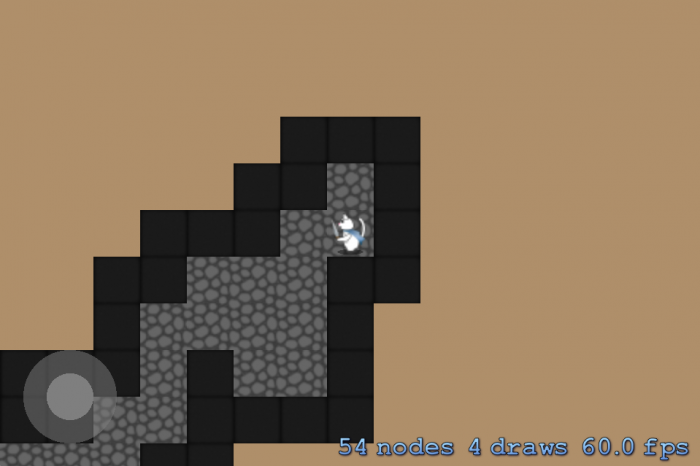Walls are kind of pointless if you can walk right through them. There are several ways to fix this problem, one of which is described in the Collisions and Collectables: How To Make a Tile-Based Game with Cocos2D 2.X, Part 2 tutorial on this site. In this tutorial you will do it a bit differently by using the build-in physics engine in Sprite Kit. Everyone likes new tech, after all.

## Procedural Collision Handling: Theory

There are many ways you could turn wall tiles into collision objects. The most obvious is to add a `physicsBody` to each wall tile, but that is not the most efficient solution. Another way, as described by Steffen Itterheim, is to use the Moore Neighborhood algorithm, but that is a tutorial in its own right.

Instead, you will implement a fairly simple method where connected wall segments are combined into a single collision object. Figure 4 illustrates this method.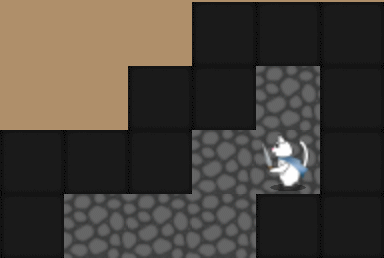Figure 4: Using a very simple method, the walls are turned into batched collision wall objects.

The method will iterate over all tiles in the map using the following logic:

1. Starting at (0,0), iterate the tile grid until you find a wall tile.
2. When you find a wall tile, mark the tile grid position. This is the starting point for the collision wall.
3. Move to the next tile in the grid. If this is also a wall tile, then increase the number of tiles in the collision wall by 1.
4. Continue step 3 until you reach a non-wall tile or the end of the row.
5. When you reach a non-tile or the end of the row, create a collision wall from the starting point with a size of the number of tiles in the collision wall.
6. Start the iteration again, go back to step 2 and repeat until you've turned all wall tiles in the grid into collision walls.

Note: The method described here is very basic and could be optimized further. For instance, you could iterate the map both horizontally and vertically. Iterating the map horizontally would omit all collision walls that are the size of one tile. You would then pick these up when iterating the map vertically, further decreasing the number of collision objects, which is always a good thing.

It's time to put theory into practice.

## Procedural Collision Handling: Practice

Look at `initWithSize:` in MyScene.m and see that the code to activate the physics engine is already in the starter project. Since Ray did an excellent job explaining how to set up the physics engine in the Sprite Kit for Beginners tutorial, I'll only explain it here in the context of procedural level generation.

When the code creates the `physicsBody` of the player object, it sets it to collide with walls by adding the `CollisionTypeWall` to the `collisionBitMask`. That way, the physics engine will automatically bounce the player off any wall objects.

However, when you created the walls in `generateWalls`, you didn't create them as physics objects – only as simple `SKSpriteNode`s. Hence, when you build and run the game the player will not collide with the walls.

You're going to simplify wall collision object creation by adding a helper method. Open Map.m and add the following code:

```// Add at the top of the file together with the other #import statements
#import "MyScene.h"

// Add with other methods
- (void) addCollisionWallAtPosition:(CGPoint)position withSize:(CGSize)size
{
SKNode *wall = [SKNode node];

wall.position = CGPointMake(position.x + size.width * 0.5f - 0.5f * self.tileSize,
position.y - size.height * 0.5f + 0.5f * self.tileSize);
wall.physicsBody = [SKPhysicsBody bodyWithRectangleOfSize:size];
wall.physicsBody.dynamic = NO;

}
```

This method creates and adds an `SKNode` to the map with the passed position and size. It then creates a non-moveable physics body for the node the size of the node, and ensures that the physics engine performs collision handling when the player collides with the node.

It's time to implement the collision wall generation. Add the following method:

```- (void) generateCollisionWalls
{
for ( NSInteger y = 0; y < self.tiles.gridSize.height; y++ )
{
CGFloat startPointForWall = 0;
CGFloat wallLength = 0;
for ( NSInteger x = 0; x <= self.tiles.gridSize.width; x++ )
{
CGPoint tileCoordinate = CGPointMake(x, y);
// 1
if ( [self.tiles tileTypeAt:tileCoordinate] == MapTileTypeWall )
{
if ( startPointForWall == 0 && wallLength == 0 )
{
startPointForWall = x;
}
wallLength += 1;
}
// 2
else if ( wallLength > 0 )
{
CGPoint wallOrigin = CGPointMake(startPointForWall, y);
CGSize wallSize = CGSizeMake(wallLength * self.tileSize, self.tileSize);
withSize:wallSize];
startPointForWall = 0;
wallLength = 0;
}
}
}
}
```

Here you perform the six steps described earlier.

1. You iterate through each row until you find a wall tile. You set a starting point (tile coordinate pair) for the collision wall and then increase the `wallLength` by one. Then you move to the next tile. If this is also a wall tile, you repeat these steps.
2. If the next tile is not a wall tile, you calculate the size of the wall in points by multiplying the tile size, and you convert the starting point into world coordinates. By passing the starting point (as world coordinates in pixels) and size (in pixels), you generate a collision wall using the `addCollisionWallAtPosition:withSize:` helper method you added above.

Go to `generate` in Map.m and add the following line of code after `[self generateTiles]` to ensure the game generates collision walls when it generates a tile map:

```
[self generateCollisionWalls];
```

Build and run. Now the cat is stuck within the walls. The only way out is to find the exit – or is it?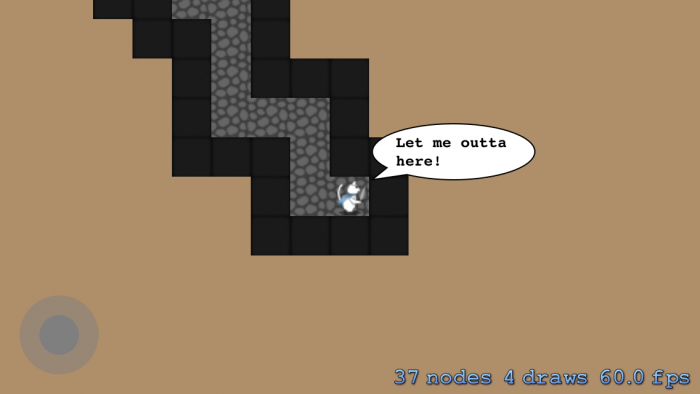## Where to Go from Here?

You've earned a basic understanding of how to generate procedural levels in your game. Here is the full source code for the first part of the tutorial.

In the second part of this tutorial, you will extend the map generation code even further by adding rooms. You'll also make map generation more controllable by adding several properties that will influence the process.

If you have any comments or suggestions related to this tutorial, please join the forum discussion below.